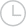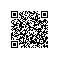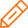# javascript中如何将长数字格式化，如"10000"变成"10,000"或"10 000"-问答-阿里云开发者社区-阿里云

## javascript中如何将长数字格式化，如"10000"变成"10,000"或"10 000"a1234566782016-03-10 18:32:451631

JavaScript 前端开发取消 提交回答

• 造物者
2019-07-17 18:58:09
``````var num = 10000;
num = num.toLocaleString();``````
0 0
• a123456678
2019-07-17 18:58:09

PHP里头有number_format函数可以对数字进行格式化，然后我找了一个JS版的number_format函数，感觉还不错。
但是我觉得这个太强大了，只是要加个逗号什么的没必要，就自己写了一个。JS新手，用PHP的思路写的JS，可能不是最好的，不过确定是可以用的，希望能帮到你。

``````function number_format(num, format) {
if(num.length <= 3) return num;
if (num.length % 3 == 0) {
count = num.length/3;
}else{
count = (num.length-num.length%3)/3;
}
var text = '';
for(i=0;i<count;i++) {
if((count-i-1)*3+num.length%3!=0) {
text=format+num.slice((count-i-1)*3+num.length%3,(count-i-1)*3+num.length%3+3)+text;
}else {
text=num.slice((count-i-1)*3+num.length%3,(count-i-1)*3+num.length%3+3)+text;
}
}
return num.slice(0,num.length % 3)+text;
}``````
0 0添加回答使用钉钉扫一扫加入圈子
+ 订阅